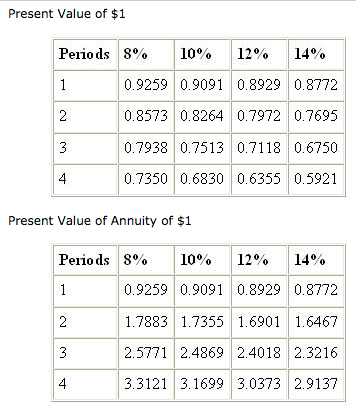# Problem: What is the selling price (to the nearest dollar) of 4-year bonds with a par value of $200,000 and an annual coupon rate of 8% that are sold when the market rate of interest is 12%? A)$180,093 B) $175,697 C)$199,994 D) $200,000 ###### FREE Expert Solution ###### Problem Details What is the selling price (to the nearest dollar) of 4-year bonds with a par value of$200,000 and an annual coupon rate of 8% that are sold when the market rate of interest is 12%?

A) $180,093 B)$175,697

C) $199,994 D)$200,000Frequently Asked Questions

What scientific concept do you need to know in order to solve this problem?

Our tutors have indicated that to solve this problem you will need to apply the Using Time Value of Money Tables concept. You can view video lessons to learn Using Time Value of Money Tables. Or if you need more Using Time Value of Money Tables practice, you can also practice Using Time Value of Money Tables practice problems.

What is the difficulty of this problem?

Our tutors rated the difficulty ofWhat is the selling price (to the nearest dollar) of 4-year ...as high difficulty.